## Understand how the graph of a parabola is related to its quadratic function

The general form of a quadratic function presents the function in the form

$f\left(x\right)=a{x}^{2}+bx+c$

where ab, and c are real numbers and $a\ne 0.$ If $a>0,$ the parabola opens upward. If $a<0,$ the parabola opens downward. We can use the general form of a parabola to find the equation for the axis of symmetry.

The axis of symmetry is defined by $x=-\frac{b}{2a}.$ If we use the quadratic formula, $x=\frac{-b\pm \sqrt{{b}^{2}-4ac}}{2a},$ to solve $a{x}^{2}+bx+c=0$ for the x-intercepts, or zeros, we find the value of x halfway between them is always $x=-\frac{b}{2a},$ the equation for the axis of symmetry.

Figure 4 shows the graph of the quadratic function written in general form as $y={x}^{2}+4x+3.$ In this form, $a=1,\text{ }b=4,$ and $c=3.$ Because $a>0,$ the parabola opens upward. The axis of symmetry is $x=-\frac{4}{2\left(1\right)}=-2.$ This also makes sense because we can see from the graph that the vertical line $x=-2$ divides the graph in half. The vertex always occurs along the axis of symmetry. For a parabola that opens upward, the vertex occurs at the lowest point on the graph, in this instance, $\left(-2,-1\right).$ The x-intercepts, those points where the parabola crosses the x-axis, occur at $\left(-3,0\right)$ and $\left(-1,0\right).$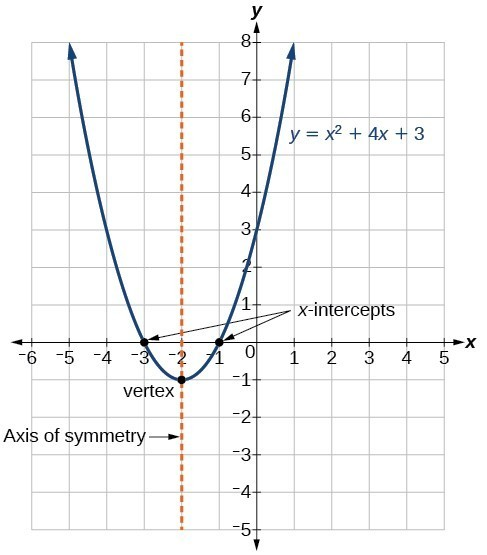Figure 4

The standard form of a quadratic function presents the function in the form

$f\left(x\right)=a{\left(x-h\right)}^{2}+k$

where $\left(h,\text{ }k\right)$ is the vertex. Because the vertex appears in the standard form of the quadratic function, this form is also known as the vertex form of a quadratic function.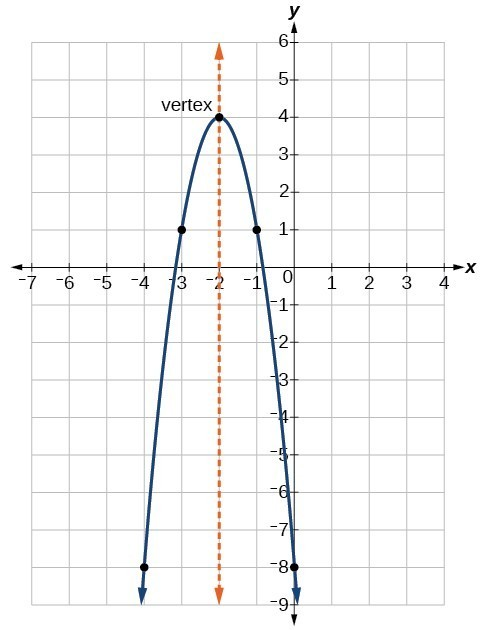Figure 5

As with the general form, if $a>0,$ the parabola opens upward and the vertex is a minimum. If $a<0,$ the parabola opens downward, and the vertex is a maximum. Figure 5 is the graph of the quadratic function written in standard form as $y=-3{\left(x+2\right)}^{2}+4.$ Since $x-h=x+2$ in this example, $h=-2.$ In this form, $a=-3,\text{ }h=-2,$ and $k=4.$ Because $a<0,$ the parabola opens downward. The vertex is at $\left(-2,\text{ 4}\right).$

The standard form is useful for determining how the graph is transformed from the graph of $y={x}^{2}.$ Figure 6 is the graph of this basic function.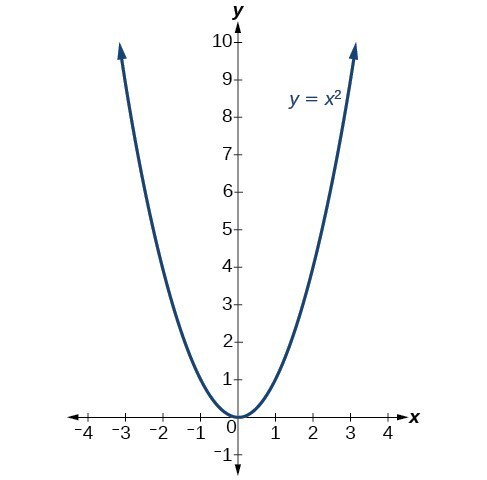Figure 6

If $k>0,$ the graph shifts upward, whereas if $k<0,$ the graph shifts downward. In Figure 5, $k>0,$ so the graph is shifted 4 units upward. If $h>0,$ the graph shifts toward the right and if $h<0,$ the graph shifts to the left. In Figure 5, $h<0,$ so the graph is shifted 2 units to the left. The magnitude of a indicates the stretch of the graph. If $|a|>1,$ the point associated with a particular x-value shifts farther from the x-axis, so the graph appears to become narrower, and there is a vertical stretch. But if $|a|<1,$ the point associated with a particular x-value shifts closer to the x-axis, so the graph appears to become wider, but in fact there is a vertical compression. In Figure 5, $|a|>1,$ so the graph becomes narrower.

The standard form and the general form are equivalent methods of describing the same function. We can see this by expanding out the general form and setting it equal to the standard form.

$\begin{cases}a{\left(x-h\right)}^{2}+k=a{x}^{2}+bx+c\hfill \\ a{x}^{2}-2ahx+\left(a{h}^{2}+k\right)=a{x}^{2}+bx+c\hfill \end{cases}$

For the linear terms to be equal, the coefficients must be equal.

$-2ah=b,\text{ so }h=-\frac{b}{2a}.$

This is the axis of symmetry we defined earlier. Setting the constant terms equal:

$\begin{cases}a{h}^{2}+k=c\hfill \\ \text{ }k=c-a{h}^{2}\hfill \\ \text{ }=c-a-{\left(\frac{b}{2a}\right)}^{2}\hfill \\ \text{ }=c-\frac{{b}^{2}}{4a}\hfill \end{cases}$

In practice, though, it is usually easier to remember that k is the output value of the function when the input is h, so $f\left(h\right)=k.$

### A General Note: Forms of Quadratic Functions

A quadratic function is a function of degree two. The graph of a quadratic function is a parabola. The general form of a quadratic function is $f\left(x\right)=a{x}^{2}+bx+c$ where a, b, and c are real numbers and $a\ne 0.$

The standard form of a quadratic function is $f\left(x\right)=a{\left(x-h\right)}^{2}+k.$

The vertex $\left(h,k\right)$ is located at

$h=-\frac{b}{2a},\text{ }k=f\left(h\right)=f\left(\frac{-b}{2a}\right).$

### How To: Given a graph of a quadratic function, write the equation of the function in general form.

1. Identify the horizontal shift of the parabola; this value is h. Identify the vertical shift of the parabola; this value is k.
2. Substitute the values of the horizontal and vertical shift for h and k. in the function $f\left(x\right)=a{\left(x-h\right)}^{2}+k.$
3. Substitute the values of any point, other than the vertex, on the graph of the parabola for x and $f\left(x\right).$
4. Solve for the stretch factor, |a|.
5. Expand and simplify to write in general form.

### Example 2: Writing the Equation of a Quadratic Function from the Graph

Write an equation for the quadratic function g in the graph below as a transformation of $f\left(x\right)={x}^{2},$ and then expand the formula, and simplify terms to write the equation in general form.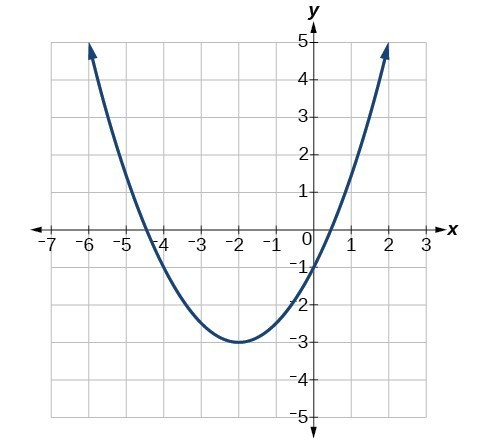Figure 7

### Solution

We can see the graph of g is the graph of $f\left(x\right)={x}^{2}$ shifted to the left 2 and down 3, giving a formula in the form $g\left(x\right)=a{\left(x+2\right)}^{2}-3.$

Substituting the coordinates of a point on the curve, such as $\left(0,-1\right),$ we can solve for the stretch factor.

$\begin{cases}-1=a{\left(0+2\right)}^{2}-3\hfill \\ \text{ }2=4a\hfill \\ \text{ }a=\frac{1}{2}\hfill \end{cases}$

In standard form, the algebraic model for this graph is $\left(g\right)x=\frac{1}{2}{\left(x+2\right)}^{2}-3.$

To write this in general polynomial form, we can expand the formula and simplify terms.

$\begin{cases}g\left(x\right)=\frac{1}{2}{\left(x+2\right)}^{2}-3\hfill \\ \text{ }=\frac{1}{2}\left(x+2\right)\left(x+2\right)-3\hfill \\ \text{ }=\frac{1}{2}\left({x}^{2}+4x+4\right)-3\hfill \\ \text{ }=\frac{1}{2}{x}^{2}+2x+2 - 3\hfill \\ \text{ }=\frac{1}{2}{x}^{2}+2x - 1\hfill \end{cases}$

Notice that the horizontal and vertical shifts of the basic graph of the quadratic function determine the location of the vertex of the parabola; the vertex is unaffected by stretches and compressions.

### Analysis of the Solution

We can check our work using the table feature on a graphing utility. First enter $\text{Y1}=\frac{1}{2}{\left(x+2\right)}^{2}-3.$ Next, select $\text{TBLSET,}$ then use $\text{TblStart}=-6$ and $\Delta \text{Tbl = 2,}$ and select $\text{TABLE}\text{.}$

 x –6 –4 –2 0 2 y 5 –1 –3 –1 5

The ordered pairs in the table correspond to points on the graph.

### Try It 1

A coordinate grid has been superimposed over the quadratic path of a basketball in the picture below. Find an equation for the path of the ball. Does the shooter make the basket?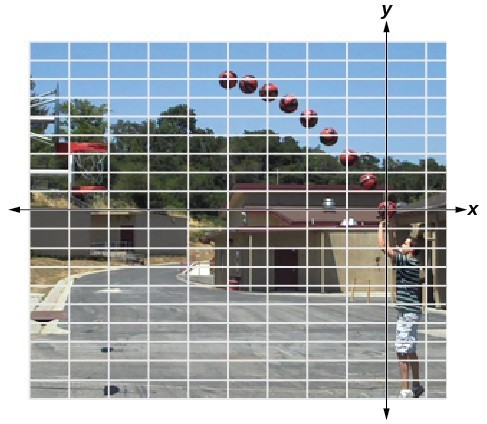Figure 8. (credit: modification of work by Dan Meyer)

Solution

### How To: Given a quadratic function in general form, find the vertex of the parabola.

1. Identify ab, and c.
2. Find h, the x-coordinate of the vertex, by substituting a and b into $h=-\frac{b}{2a}.$
3. Find k, the y-coordinate of the vertex, by evaluating $k=f\left(h\right)=f\left(-\frac{b}{2a}\right).$

### Example 3: Finding the Vertex of a Quadratic Function

Find the vertex of the quadratic function $f\left(x\right)=2{x}^{2}-6x+7.$ Rewrite the quadratic in standard form (vertex form).

### Solution

The horizontal coordinate of the vertex will be at

$\begin{cases}h=-\frac{b}{2a}\hfill \\ \text{ }=-\frac{-6}{2\left(2\right)}\hfill \\ \text{ }=\frac{6}{4}\hfill \\ \text{ }=\frac{3}{2}\hfill \end{cases}$

The vertical coordinate of the vertex will be at

$\begin{cases}k=f\left(h\right)\hfill \\ \text{ }=f\left(\frac{3}{2}\right)\hfill \\ \text{ }=2{\left(\frac{3}{2}\right)}^{2}-6\left(\frac{3}{2}\right)+7\hfill \\ \text{ }=\frac{5}{2}\hfill \end{cases}$

Rewriting into standard form, the stretch factor will be the same as the $a$ in the original quadratic.

$\begin{cases}f\left(x\right)=a{x}^{2}+bx+c\hfill \\ f\left(x\right)=2{x}^{2}-6x+7\hfill \end{cases}$

Using the vertex to determine the shifts,

$f\left(x\right)=2{\left(x-\frac{3}{2}\right)}^{2}+\frac{5}{2}$

### Analysis of the Solution

One reason we may want to identify the vertex of the parabola is that this point will inform us where the maximum or minimum value of the output occurs, (k), and where it occurs, (x).

### Try It 2

Given the equation $g\left(x\right)=13+{x}^{2}-6x,$ write the equation in general form and then in standard form.

Solution

## Finding the Domain and Range of a Quadratic Function

Any number can be the input value of a quadratic function. Therefore, the domain of any quadratic function is all real numbers. Because parabolas have a maximum or a minimum point, the range is restricted. Since the vertex of a parabola will be either a maximum or a minimum, the range will consist of all y-values greater than or equal to the y-coordinate at the turning point or less than or equal to the y-coordinate at the turning point, depending on whether the parabola opens up or down.

### A General Note: Domain and Range of a Quadratic Function

The domain of any quadratic function is all real numbers.

The range of a quadratic function written in general form $f\left(x\right)=a{x}^{2}+bx+c$ with a positive a value is $f\left(x\right)\ge f\left(-\frac{b}{2a}\right),$ or $\left[f\left(-\frac{b}{2a}\right),\infty \right);$ the range of a quadratic function written in general form with a negative a value is $f\left(x\right)\le f\left(-\frac{b}{2a}\right),$ or $\left(-\infty ,f\left(-\frac{b}{2a}\right)\right].$

The range of a quadratic function written in standard form $f\left(x\right)=a{\left(x-h\right)}^{2}+k$ with a positive a value is $f\left(x\right)\ge k;$ the range of a quadratic function written in standard form with a negative a value is $f\left(x\right)\le k.$

### How To: Given a quadratic function, find the domain and range.

1. Identify the domain of any quadratic function as all real numbers.
2. Determine whether a is positive or negative. If a is positive, the parabola has a minimum. If a is negative, the parabola has a maximum.
3. Determine the maximum or minimum value of the parabola, k.
4. If the parabola has a minimum, the range is given by $f\left(x\right)\ge k,$ or $\left[k,\infty \right).$ If the parabola has a maximum, the range is given by $f\left(x\right)\le k,$ or $\left(-\infty ,k\right].$

### Example 4: Finding the Domain and Range of a Quadratic Function

Find the domain and range of $f\left(x\right)=-5{x}^{2}+9x - 1.$

### Solution

As with any quadratic function, the domain is all real numbers.

Because a is negative, the parabola opens downward and has a maximum value. We need to determine the maximum value. We can begin by finding the x-value of the vertex.

$\begin{cases}h=-\frac{b}{2a}\hfill \\ \text{ }=-\frac{9}{2\left(-5\right)}\hfill \\ \text{ }=\frac{9}{10}\hfill \end{cases}$

The maximum value is given by $f\left(h\right).$

$\begin{cases}f\left(\frac{9}{10}\right)=5{\left(\frac{9}{10}\right)}^{2}+9\left(\frac{9}{10}\right)-1\hfill \\ \text{ }=\frac{61}{20}\hfill \end{cases}$

The range is $f\left(x\right)\le \frac{61}{20},$ or $\left(-\infty ,\frac{61}{20}\right].$

### Try It 3

Find the domain and range of $f\left(x\right)=2{\left(x-\frac{4}{7}\right)}^{2}+\frac{8}{11}.$

Solution